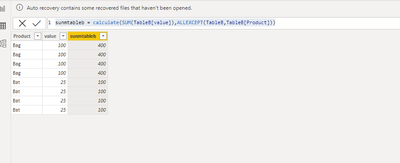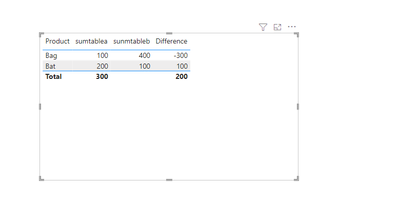cancel
Showing results for
Did you mean:Frequent Visitor

## Creating a measure using two different column from different table

Hi There,

I am trying to create a calculated measure where i am taking difference from two columns(one is calculated measure and one is calculated column) which is avaiable in two different tables and they have Many to Many relationship .

 Product Table A (Amount in Rs) Table B (Amount in Rs) Difference Bag 100 400 300 Bat 200 100 -100

Desrire Result i am looking for is column name "Difference"

Can anyone help me how to achieve this ??

Thanks ,

Ashish

1 ACCEPTED SOLUTIONCommunity Support

Hi  @ash_cool10 ,

Refer the below:

``sumtablea = CALCULATE(SUM(TableA[value]),ALLEXCEPT(TableA,TableA[Product]))````sunmtableb = calculate(SUM(TableB[value]),ALLEXCEPT(TableB,TableB[Product]))``Relationship:Difference:

``Difference = [sumtablea]-LOOKUPVALUE(TableB[sunmtableb],TableB[Product],MAX(TableA[Product]))``

Outputresult:Best Regards

Lucien

5 REPLIES 5Community Support

Hi  @ash_cool10 ,

Refer the below:

``sumtablea = CALCULATE(SUM(TableA[value]),ALLEXCEPT(TableA,TableA[Product]))````sunmtableb = calculate(SUM(TableB[value]),ALLEXCEPT(TableB,TableB[Product]))``Relationship:Difference:

``Difference = [sumtablea]-LOOKUPVALUE(TableB[sunmtableb],TableB[Product],MAX(TableA[Product]))``

Outputresult:Best Regards

LucienFrequent Visitor

Hi Lucien,

Thank you so much for your support. I tried and i got the result .

Regards,

AshishSuper User

@ash_cool10 use the same process that you do to create a calculated column and a measure

1. first create a calculated columns

3. then create your measure to calc difference between 1. calculated column 2. measure

in case you face any challenge, please sample data to create above calc

Proud to be a Super User!Frequent Visitor

Hi @negi007,

I tried creating the same but nothing is working out.

It gives error "a table of multiple values was supplied where a single value was expected" when i used Lookup value fucntion to call Calculated COlumn value inn Table 1.

Can you please elaborate some more?

Thanks.

ASuper User

@ash_cool10  why do you need to use lookup function. both tables are already linked to each other through relationship you have created as mentioned by you.

let say you first create a calculated column like

1. Profit_Est = Orders[Profit]*1.5

then you create a measure in the second table like below
2. Returns = sum(Returns[value])

then you create a measure like below
Profit_Returns = SUM(Orders[Profit_Est])-[Returns]

i hope it can direct you in the right direction. for exact help would it be possible for you to share your pbix file or data in text format. thanks

Proud to be a Super User!